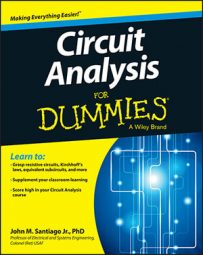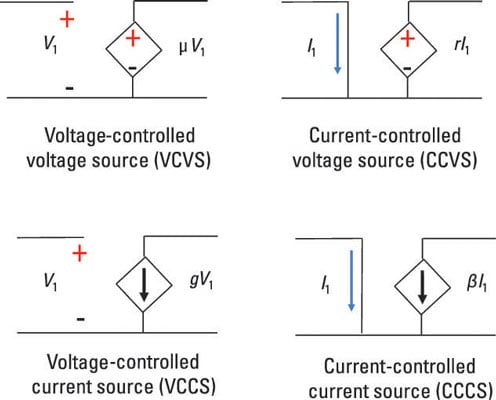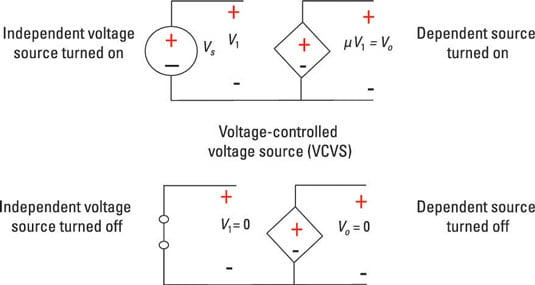##### Circuit Analysis For DummiesDependent sources are used to model transistors and the operational amplifier IC. A dependent source is a voltage or current source controlled by either a voltage or a current at the input side of the device model. The dependent source drives the output side of the circuit.

Dependent sources are usually associated with components (or devices) requiring power to operate correctly. These components are considered active devices because they require power to work; circuits using these devices are called active circuits. Active devices such as transistors perform amplification, allowing you to do things like crank up the volume of your music.

When you’re dealing with active devices operating in a linear mode, the relationship between the input and output behavior is directly proportional. That is, the bigger the input, the bigger the output. Mathematically for a given input x, you have an output y with a gain amplification of G: y = Gx.

The constant or gain G is greater than 1 for active circuits (think steroids) and less than 1 for passive circuits (think wimpy). In other engineering applications, technical terms for G include scale factor, scalar multiplier, proportionality constant, and weight factor.

## Classify the types of dependent sources

Modeling active devices requires the use of dependent sources, and four types of dependent sources exist as shown here:• Voltage-controlled voltage source (VCVS): A voltage across the input terminals controls a dependent voltage source at the output port.

• Current-controlled voltage source (CCVS): A current flowing through the input terminals controls a dependent voltage source.

• Voltage-controlled current source (VCCS): Now account for a dependent current source at the output terminals. With a voltage across the input, you can control the amount of current output.

• Current-controlled current source (CCCS): Can you guess the last type of dependent source? That’s right — with a current flowing through the input port, you can control a dependent current source at the output port.

Diagrams use the diamond shape for dependent sources to distinguish them from independent sources, which use a circle.

The output of a linear dependent source is proportional to the input voltage or current controlling the source output. In the sample circuit, the proportionality constants or gains are given as μ, r, g, and β:

• You can think of μ in the VCVS dependent source as voltage gain because it’s the ratio of the voltage output to the voltage input.

• In the CCVS dependent source, the proportionality constant r is called the transresistance because its input-output relationship takes the form of Ohm’s law: v = iR.

• Similarly, the VCCS dependent source has a proportionality constant g, called the transconductance, following a variation of Ohm’s law: i = Gv (where the conductance G = 1/R).

• For the CCCS dependent source, you can think of the proportionality constant β as the current gain because it’s the ratio of current output to current input.

So when the type of input matches the type of output, the proportionality constant gives you the current or voltage gain. When they differ, the input-output relationship stems from Ohm’s law.

## Recognize the relationship between dependent and independent sources

You can turn off an independent voltage source by replacing it with a short circuit having zero resistance, and you can turn off an independent current source by replacing it with an open circuit. But you can’t just turn dependent sources on or off. Because dependent sources rely on voltage or current from an independent source on the input side, turning off an independent source turns off a dependent source.

This illustrates the interplay between the independent source and the dependent source.The top diagram shows that when an independent source is turned on, the dependent source is turned on. The bottom diagram shows that when an independent source is turned off, the dependent source is turned off.